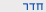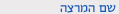שנה"ל תש"ףאנליזה על יריעות
Analysis on Manifolds
0366-3115
מדעים מדויקים | מתמטיקה
קבוצה 01סמ'  ב'1200-1300007 שרייבר מתמטישיעור ות פרופ אלסקר סמיון
סמ'  ב'1300-1500105 פיזיקה-שנקרשיעור ות
ש"ס:  3.0

סילבוס מקוצר

This is a course for undergraduate and graduate mathematics and physics students. Its purpose is to give an introduction to the theory of manifolds -- topological spaces which locally look as the usual Euclidean space but may have a complicated global shape. Manifolds play a basic role in modern mathematics and mathematical physics.

We will cover the following topics:

1) Manifolds (definition, examples, constructions).
2) Topological properties of manifolds and partition of unity.
3) Tangent space and the differential.
4) Immersions, submersions, and submanifolds.
5) Embedding and Whitney's theorem.
6) Vector fields and flows (Lie bracket & Lie derivative).
7) Orientation.
8) Degree of a map (and applications: Brouwer fixed point, Borsuk-Ulam, the "hairy ball" theorem, etc.).
9) Differential forms.
10) Integration on manifolds (Stokes theorem).
11) de Rham cohomology.
If time permits:
12) Vector bundles, parallel transport, connection and covariant differentiation, curvature.מדעים מדויקים | מתמטיקה
0366-3115-01 אנליזה על יריעות
Analysis on Manifolds
שנה"ל תש"ף | סמ'  ב' | פרופ אלסקר סמיון

סילבוס מפורט/דף מידע

Part I. Manifolds and maps
1. Manifolds, submanifolds, tangent bundle, boundary, orientation. 2. Homotopy and applications. Brower's fixed point theorem.
3. Degree of a map as a homotopy invariant.
4. Euler characteristic and vector fields.
Part II. Differential forms
1. Linear and local theory: exterior product and differential.
2. The Lie derivative.
3. Integration, Stokes theorem.
4. Applications: Linking number and Gauss integral, Gauss-Bonnet theorem for a surface in the 3-space, the residue formula.
5. Degree of a map revisited.
Part III. Introduction to De Rham cohomology
Prerequisites: Linear Algebra, Calculus, Ordinary Diff. Equations1-Some knowledge of basic notions of General Topology and/or Differential Geometry could be useful but is not obligatory (all necessary preliminaries will be reminded).

להצהרת הנגישות